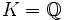# Galois-realizable group

This term associates to every field, a corresponding group property. In other words, given a field, every group either has the property with respect to that field or does not have the property with respect to that field

This term is related to: Galois theory
View other terms related to Galois theory | View facts related to Galois theory

## Definition

### Definition with symbols

A group$G$ is said to be Galois-realizable over a field$K$ if there exists a normal field extension$L$ of$K$ such that$G$ is the Galois group of$L/K$.

If no field is specified, we assume that the field is$K = \mathbb{Q}$, the field of rational numbers.

The inverse Galois problem essentially asks for which finite groups can be expressed as the Galois group of a field extension of$\mathbb{Q}$.

### Definition in terms of the universal Galois group

A group$G$ is Galois-realizable over a field$K$ if it is the quotient of the universal Galois group of$K$ by a normal subgroup which is closed under the naturally given Krull topology.

## Relation with other properties

### Weaker properties

The following properties are known to be weaker than the property of being Galois-realizable over$\mathbb{Q}$: